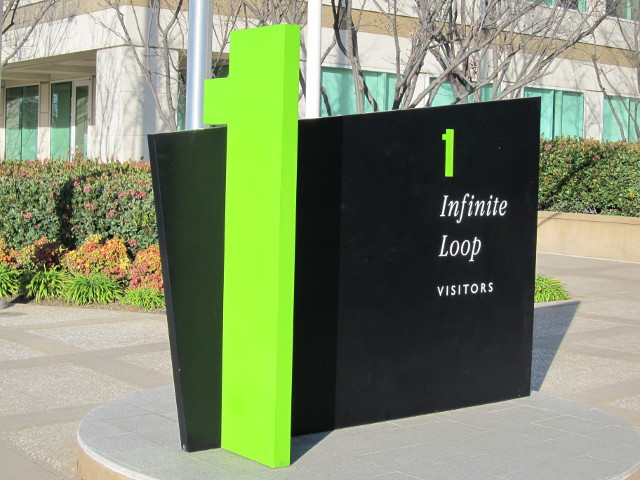# No end in sight

yourbasic.org/golangWhy does this loop run forever?

``````var b byte
for b = 250; b <= 255; b++ {
fmt.Printf("%d %c\n", b, b)
}``````

After the `b == 255` iteration, `b++` is executed. This overflows (since the maximum value for a byte is 255) and results in `b == 0`. Therefore `b <= 255` still holds and the loop restarts from 0.

For unsigned integer values, the operations +, -, *, and << are computed modulo 2n, where n is the bit width of the unsigned integer type.

For signed integers, the operations +, -, *, and << may legally overflow and the resulting value exists and is deterministically defined by the signed integer representation, the operation, and its operands. No exception is raised as a result of overflow. The Go Programming Language Specification: Arithmetic operators

If we use the standard loop idiom with a strict inequality, the compiler will catch the bug.

``````var b byte
for b = 250; b < 256; b++ {
fmt.Printf("%d %c\n", b, b)
}
``````
``````../main.go:2:17: constant 256 overflows byte
``````

One solution is to use a wider data type, such as an `int`.

``````for i := 250; i < 256; i++ {
fmt.Printf("%d %c\n", i, i)
}
``````
``````250 ú
251 û
252 ü
253 ý
254 þ
255 ÿ
``````

Another option is to put the termination test at the end of the loop.

``````for b := byte(250); ; b++ {
fmt.Printf("%d %c\n", b, b)
if b == 255 {
break
}
}
``````
``````250 ú
251 û
252 ü
253 ý
254 þ
255 ÿ
``````